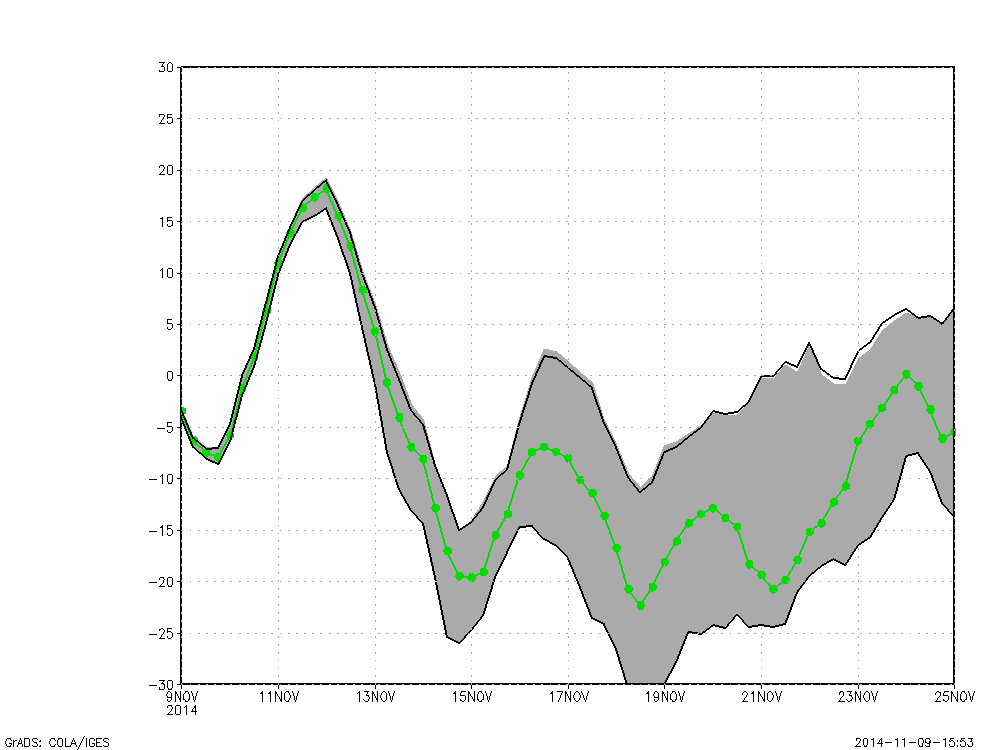## Monday, November 17, 2014

### Tutorial: Fill between; how to shade area on line graphs

Sometimes when plotting data on simple line graph it is useful to use colors to fill between different curves.  For example, when plotting anomalies it may be helpful to fill the below zero values blue, and the above zero values red (see below figure).  This tutorial will show you how to use the gxout option 'linefill' to fill areas on your x/y graph.500mb height anomaly.  Negative values filled blue.

'set gxout linefill' requires two input values, and will fill the space between these value.  It has been used before in previous tutorials here to help you fill in topography for vertical cross sections (see: here for this example).  We will begin by setting up our file and location.  I've been interested in ensemble forecasting recently, so we'll work with the GFS ensemble data.

'set display color white'
'clear'
'set mpdset hires'

'set t 1 65'
'set e 1'
'set lev 500'
'set lat 44';'set lon -72'

'set vrange -30 30'

Notice how I only have one varying dimension (time) in this tutorial.  This will allow us to plot a basic line graph, showing the 500mb height anomaly in time.  Now we are ready to move on to plot the data.  We will be plotting the ensemble mean height anomaly.  >0 => Red <0 => Blue

'set gxout linefill'
'set lfcols 2 4'
'd mean(gpa500mb/10.0,e=1,e=21);const(mean(gpa500mb/10.0,e=1,e=21),0,-a)'

The above code first sets up, then makes the plot.  First use set 'gxout linefill' to get the graphics set up correctly.  The key to getting the different colors is using the command 'set lfcols 2 4'.  What this command does, is set up your plotting environment such that any value greater than the reference value is set to red(2) and anything less than the reference value is set to blue (4).  Once our colors are set, we plot the variable, in this case the ensemble mean 500mb height (divided by 10 to get units: Decameters).

When using the linefill graphics option , you need two inputs separated by a semi-colon.  These values indicate the values that will be filled between.  In this case, the first value is the height anomaly, and the second value uses the const function to set all height anomalies = 0.  So in this case, you are plotting the height anomaly relative to the zero line.  This code will give you the image above.

We can take this one step further.  The 2nd value in the 'linefill' display does not have to be a constant, it can vary.  So to stick with the theme of ensemble prediction, we'll plot the height anomaly again, only now we will fill the + and - 1 standard deviation around the mean.GFS Ensemble mean Height anomaly and shaded Standard Deviation

The following will produce the above plot.  The method for producing the above plot is very similar to what we just discussed,  only now, instead of using the const function to set the 2nd value in the linefill display, we use two variable values.

'set lfcols 15 0'
'd mean(gpa500mb/10.0,e=1,e=21)+sqrt(ave(pow(ave(gpa500mb/10.0,e=1,e=21)-gpa500mb/10.0,2),e=1,e=21));mean(gpa500mb/10.0,e=1,e=21)-sqrt(ave(pow(ave(gpa500mb/10.0,e=1,e=21)-gpa500mb/10.0,2),e=1,e=21))'

Now we fill every value between the first (mean + 1std) and the second (mean - 1std) in gray.  Then to make the plot nicer, we throw on the ensemble mean, and we cap the standard deviation with black lines.

There is one last aspect with linefill, that I feel should be included in this tutorial, and that is how to fill the area under the curve with more than 1 color (see example below).  For this, we use similar code as above, only now we put it in a loop.  Now to do this, it works best if your variable is always greater than zero, due to the way the looping is performed.  So we are going to use total cloud cover as our variable.  Additionally, to save time, I'm only going to take a subset of times from the file, and only a subset of ensembles.

So to start, we set the range of levels that we want to shade in using bars, then we simply loop through all of the levels, use the linefill display, and simply reset the value in the const function to correspond to each level.

levs='0 10 20 30 40 50 60 70 80 90'
c=1
while(subwrd(levs,c)!='')
'set gxout linefill'
'set lfcols 'c+1' 0'
'd mean(tcdcclm,e=1,e=5);const(mean(tcdcclm,e=1,e=5),'subwrd(levs,c)',-a)'
c=c+1
endwhile

'set gxout line'
'set ccolor 3'
'set cthick 8'
'd mean(tcdcclm,e=1,e=5)'

The code above uses the subwrd command to grab the value from the "array" levs.  It breaks the loop if you run into the end of the array.  Then the code sets a new fill color, and voila, you have a multi-colored shaded plot.

So that's about all there is to the linefill function.  Hopefully after this tutorial you have a better understanding of how the linefill function works, and what some of it's capabilities are.  Below is a script with the various examples used here.